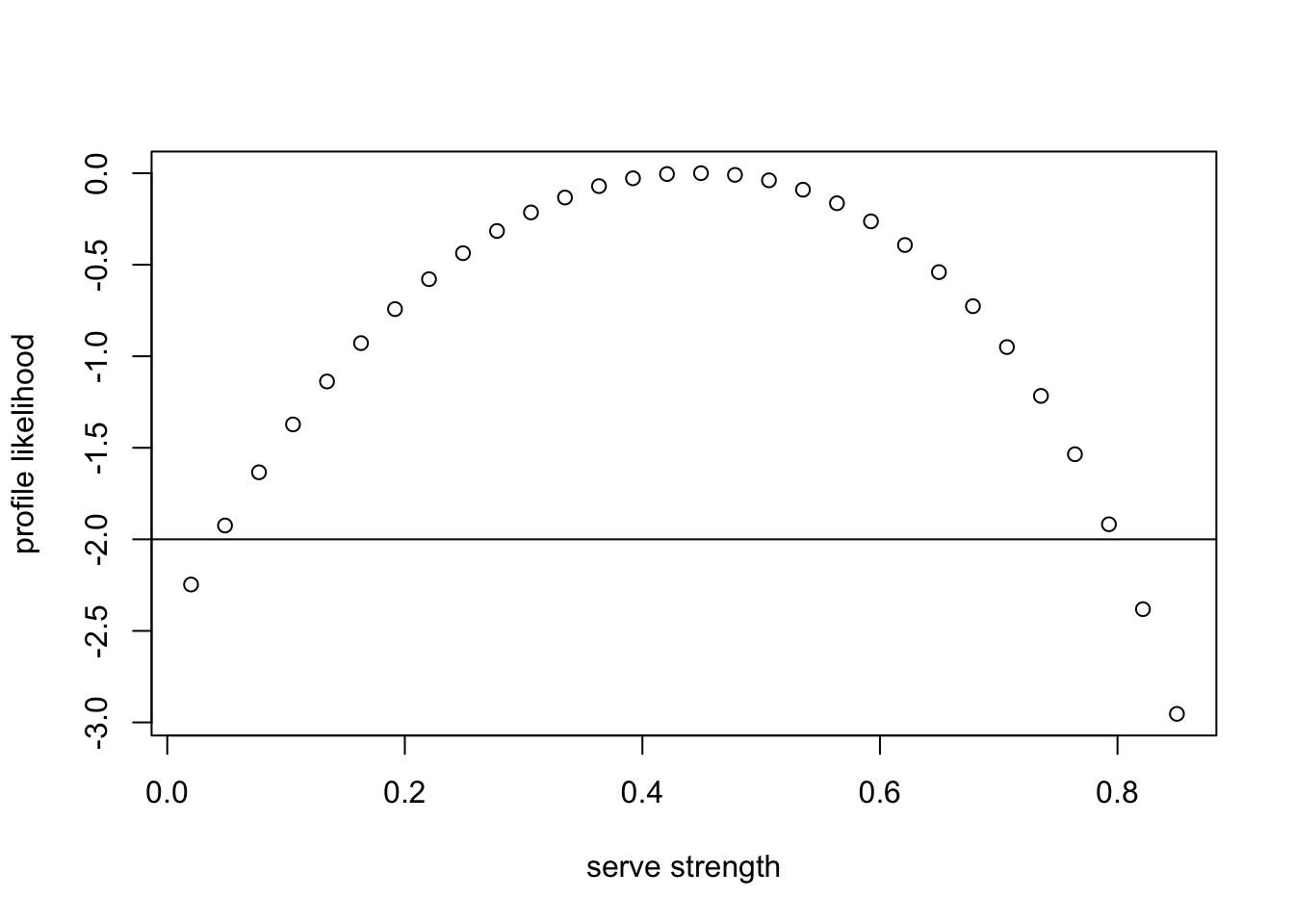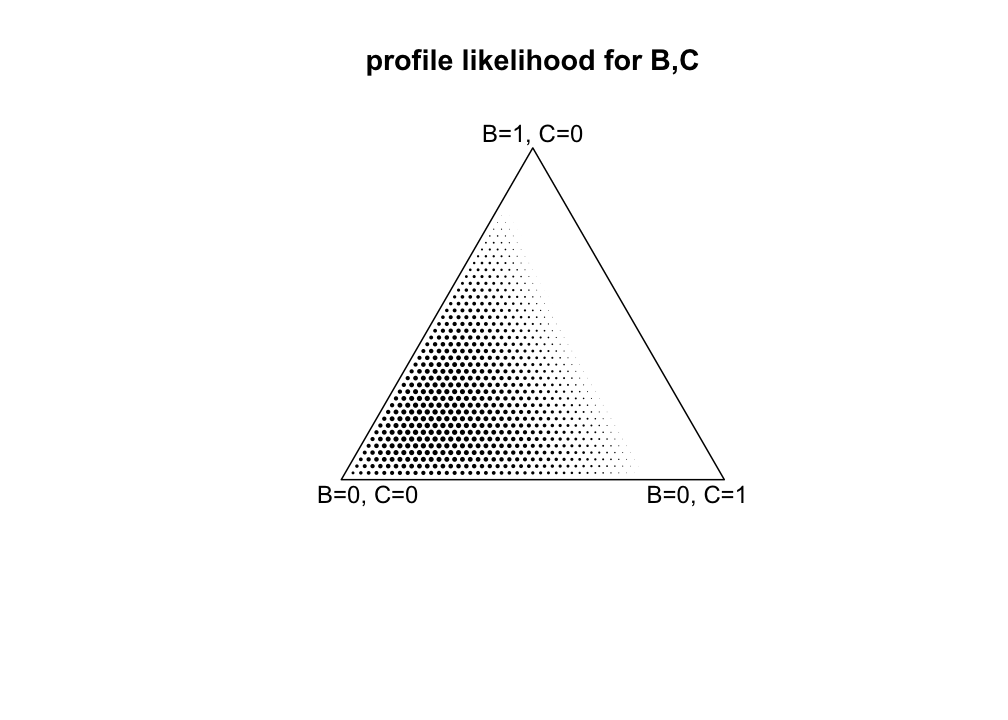# The importance of serve in table tennis.

(In this vignette, object H is created. It is identical to object table_tennis in the package; to see it type data(table_tennis) at the command line, and to see the help page, type help(table_tennis)).

Suppose we have three players, “A”,“B”, and “C” who play table tennis. We seek to quantify the effect of the serve. The scoreline is as follows:

• A vs B, A serves, 5-1
• A vs B, B serves, 1-3
• A vs C, A serves, 4-1
• A vs C, C serves, 1-2

(note that B does not play C). This dataset is easily analysed with the hyper2 package although the model implicitly assumes that the serve strength does not depend on who is serving.

library("hyper2",quietly=TRUE)

The first step is to define a hyper2 object:

H <- hyper2(pnames=c("S","a","b","c"))
H
## (S + a + b + c)^0

so the likelihood function is uniform, for the only term is raised to the zeroth power (players’ names are not capitalised in R idiom for clarity). Now we have to input the data, and we will go through the four scorelines above, one by one. Note that this approach implicitly assumes independence of scores.

### A vs B, A serves, score 5-1:

H[c("a","S")]     %<>% "+"(5  ) # a+S win 5 games
H["b"]            %<>% "+"(  1) # b wins 1 game
H[c("a","b","S")] %<>% "-"(5+1) # a+b+S play 1+5=6 games
H
## (S + a)^5 * (S + a + b)^-6 * b

The likelihood function is of the form $$\frac{\left(a+S\right)^5b}{\left(a+b+S\right)^6}$$. We can add the other observations to $$H$$:

### A vs B, B serves, score 1-3:

H[c("b","S")]     %<>% "+"(3  ) # b+S win 3 games
H["a"]            %<>% "+"(  1) # a wins 1 game
H[c("a","b","S")] %<>% "-"(3+1) # a+b+S play 1+3=4 games

### A vs C, A serves, score 4-1:

H[c("a","S")]     %<>% "+"(4  ) # a+S win 4 games
H["c"]            %<>% "+"(  1) # c wins 1 game
H[c("a","c","S")] %<>% "-"(4+1) # a+c+S play 1+4=5 games

### A vs C, C serves, score 1-2:

H["a"]            %<>% "+"(1  ) # a wins 1 game
H[c("c","S")]     %<>% "+"(  2) # c+S win 2 games
H[c("a","c","S")] %<>% "-"(1+2) # a+c+S play 1+2=3 games

## Likelihood function

For the entire dataset we have

H
## (S + a)^9 * (S + a + b)^-10 * (S + a + c)^-8 * (S + b)^3 * (S +
## c)^2 * a^2 * b * c

Here $$H$$ is a likelihood function on $$a,b,c,S$$, algebraically proportional to

$\frac{(S+a)^9(S+b)^3(S+c)^2a^2bc}{(S+a+b)^{10}(S+a+c)^8}$

# Results

We now find the unconstrained MLE:

p1 <- maxp(H)
p1
##         S         a         b         c
## 0.4504171 0.2410390 0.1445159 0.1640281

And we can find the support at this point:

maxlike_free <- loglik(H,indep(p1))
maxlike_free
##  -9.399965

Now find the MLE conditional on the serve having zero strength:

small <- 1e-4
maxlike_constrained <-
maxp(
H,
startp=c(small/2, 1/3-small/2, 1/3-small/2),
fcm=c(-1,0,0),  fcv=-small,give=TRUE
)

maxlike_constrained
## $par ##  9.999977e-05 4.413479e-01 2.941790e-01 ## ##$value
##  -12.0213
##
## $counts ## function gradient ## 491 75 ## ##$convergence
##  0
##
## $message ## NULL ## ##$outer.iterations
##  2
##
## $barrier.value ##  0.0001073469 The difference in support is: delta_support <- maxlike_free - maxlike_constrained$value
delta_support
##  2.621339

and the likelihood ratio is:

LR <- exp(delta_support)
LR
##  13.75412

The difference in support, delta_support, is about 2.62 which exceeds Edwards’s two-units-of-support criterion. We can reject the null. Alternatively, Wilks’s theorem gives us that $$\Lambda=2\log(LR)$$ is distributed as chi-squared with one degree of freedom:

Lambda <- 2*delta_support
pval <- pchisq(Lambda,df=1,lower.tail=FALSE)
pval 
##  0.02203933

Thus the tail area, pval, is about 0.022 which gives us a significant result: the serve has nonzero strength.

## Assessment of B vs C

The maximum likelihood estimate for strengths allows us to assess what might happen when B plays C (which was not observed). For convenience, here is the evaluate:

maxp(H)
##         S         a         b         c
## 0.4504171 0.2410390 0.1445159 0.1640281

If B serves, we would have $$B+S:C\simeq 0.14+0.45:0.15\equiv 59:15$$, or B wins with probability of about $$0.78$$. If C serves we have $$B:C+S\simeq 0.14:0.16+0.45\equiv 14:61$$, so B wins with a probability of about $$0.19$$.

# Profile likelihood for serve strength

We can give a profile likelihood for $$S$$ by maximizing the likelihood of $$(S,p_a,p_b,p_c)$$ subject to a particular value of $$S$$ (in addition to the unit sum constraint). The following function defines a profile likelihood function for $$S$$:

profile_likelihood <- function(S,give=FALSE){
small <- 1e-2  # cannot make this too small, optimization routine needs wiggle room
out <- maxp(
H, startp=c(S+small/2 , small,small),
give=TRUE,
fcm=rbind(c(1,0,0),c(-1,0,0)),fcv=c(S,-S-small)  # S <= p <= S+small
)
if(give){
return(out)
} else {
return(out$value) } } We can then evaluate the profile likelihood for a range of possible values of $$S$$: S_vals <- seq(from=0.02,to=0.85,len=30) # possible values for S prof_like <- sapply(S_vals,profile_likelihood) plot(S_vals, prof_like-max(prof_like),xlab="serve strength",ylab="profile likelihood") abline(h= -2)So we can see that the credible range is from about 0.07-0.8 (and in particular excludes zero). # Profile likelihood for B vs C The profile likelihood technique can be applied to the question of the strengths of players B and C, even though no competition between the players was observed: proflike <- function(bc,give=FALSE){ B <- bc C <- bc if(B+C>=1){return(NA)} objective <- function(S){ # jj <- c(S,A=1-(S+B+C),B,C) # B,C fixed loglik(H,indep(jj)) # no minus: we use maximum=T in optimize() } maxlike_constrained <- # single DoF is S [B,C given, A is fillup] optimize( # single DoF -> use optimize() not optim() f = objective, interval = c(0,1-(B+C)), maximum = TRUE ) if(give){ return(maxlike_constrained) } else{ return(maxlike_constrained$objective)
}
}

small <- 1e-5
n <- 50
p <- seq(from=small,to=1-small,length=n)
jj <- expand.grid(p,p)
support <- apply(jj,1,proflike)
support <- support-max(support,na.rm=TRUE)
supportx <- support
dim(supportx) <- c(n,n)

x <- jj[,1]+jj[,2]/2
y <- jj[,2]*sqrt(3)/2
plot(x,y,cex=(support+6)/12,
pch=16,asp=1,xlim=c(-0.1,1.1),ylim=c(-0.2,0.9),
axes=FALSE,xlab="",ylab="",main="profile likelihood for B,C")
polygon(x=c(0,1/2,1),y=c(0,sqrt(3)/2,0))
text(0.07,-0.04,'B=0, C=0')
text(0.50,+0.90,'B=1, C=0')
text(0.93,-0.04,'B=0, C=1')But what one is really interested in is the relative strength of player B and player C. We are indifferent between $$p_B=0.1,p_C=0.2$$ and $$p_B=0.3,p_C=0.6$$. To this end we need the profile likelihood curve of the log contrast, $$X=\log\left(p_B/p_C\right)$$.

logcontrast <- apply(jj,1,function(x){log(x/x)})
plot(logcontrast,support,xlim=c(-5,5),ylim=c(-4,0))
abline(h=-2)